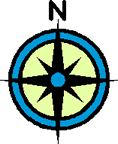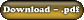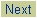Area
Objectives

To calculate the area of even and uneven boundaries on a topographic map using the squares method.Let's look at how to calculate the area of even and uneven boundaries on a topographic map.

 Area of a square Formula: Length x Width

 Calculating the area of an even boundary Example A map with a scale of 1:50 000. The length of this map is 5cm and the width is 3cm. Calculate the area represented by the map. *Convert the length and width into what they represent in reality before using the formula*. Scale 1:50 000 - therefore each cm on the map represents 0.5 km in reality Length: 5cm x 0.5 km = 2.5km Width: 3cm x 0.5 km = 1.5 km Area = Length x Width = 2.5km x 1.5km = 3.75 sq. km Calculating the area of an uneven boundary Example One Open the map of Houston, B.C.Calculate the area of Klinger Lake. Step 1: Work out the area of one grid square on the map. Scale of this map: 1:50 000, therefore each cm represents 0.5km Note: Adjust the pdf file by zooming in or out until each grid square is 2cm in length. Length: 2cm x 0.5km = 1.0km Width: 2cm X 0.5 km = 1.0km Area of One Grid Square = 1.0km x 1.0km = 1.0 sq. km Step 2: Determine the number of squares that the object occupies. Klinger Lake occupies a total of about 0.5 of a grid square. Step 3: Multiply the number of grid squares that the object occupies by the area of one grid square. Hence, 0.5 of a square x 1.0 sq. km = 0.5 sq. km which is the area of Klinger Lake. Example Two Open the map of St. John's, Newfoundland & Labrador.Calculate the area of the built up portion of the city (i.e. the pink shaded area). Step 1: Work out the area of one grid square on the map. Note: Adjust the pdf file by zooming in or out until each grid square is 2cm in length. It is the same as the previous map: 1.0 sq.km. Step 2: Determine the number of squares that the object occupies. In this situation, one could go from grid square to grid square determing how much the built up area occupies each square. i.e. 0.1 of a grid square (5866) + 0.4 (5966) + 0.7 (5967) + 0.7 (6067) + 0.03 (5868) + 0.5 (5968) + 1.0 (6068) + 0.25 (6168) + 0.2 (5869) + 0.6 (5969) + 1.0 (6069) + 0.85 (6169) + 0.2 (6269) + 0.8 (6070) + 0.85 (6170) + 0.2 (6270) + 0.2 (6071) + 0.4 (6171) = 8.98 squares Step 3: Multiply the number of grid squares that the object occupies by the area of one grid square. Hence, 8.98 squares x 1.0 sq.km = 8.98 sq. km which is the area of downtown St. John's. Note: Clearly, there is an acceptable range for the answer due to measuring error. On the government exam, this would be a multiple choice question. Hence, you would pick the answer closest to the one that you determined.

 Assignment Work 1) Using the map of St. John's, determine the area of Quidi Vidi lake. 2) Determine the area of Long Pond. 3) Determine the area on the map south of Blackhead Road and east of Maddox Cove Road. 4) Check over your answers using the answer key.©www.coolschool.ca Press thebutton on the Action menu to proceed.